# SSAT Middle Level Math : How to find the area of a rectangle

## Example Questions

1 2 3 5 Next →

### Example Question #41 : How To Find The Area Of A Rectangle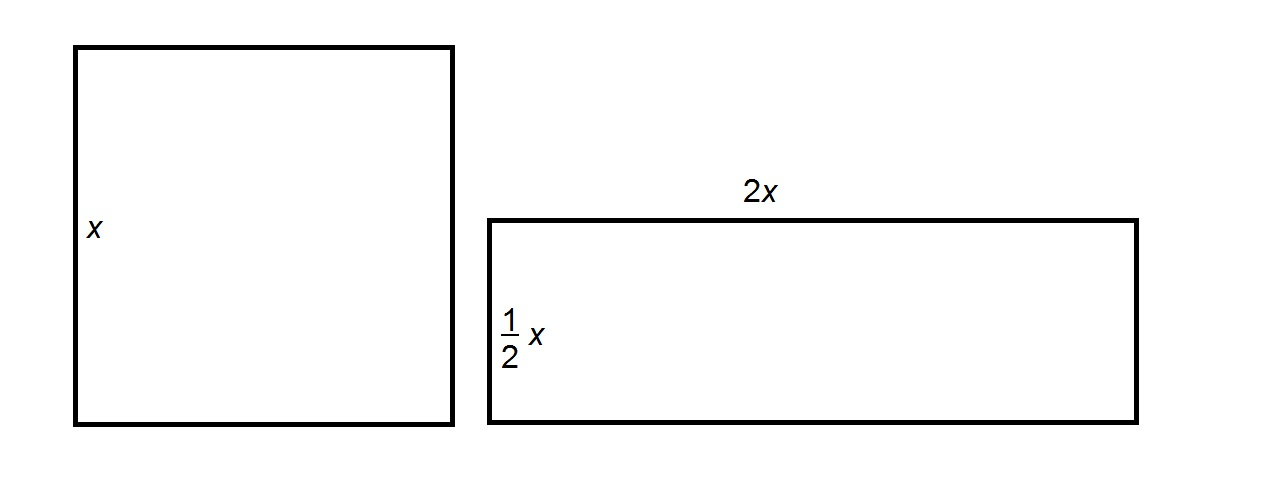Refer to the above figures. The square at left has area 160. Give the area of the rectangle at right.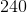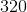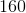Explanation:

The area of the square, whose sides have length, is the square of this sidelength, which is. The area of the rectangle is the product of the lengths of its sides; this is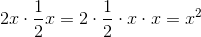The square and the rectangle have the same area, so the correct response is 160.

### Example Question #42 : How To Find The Area Of A Rectangle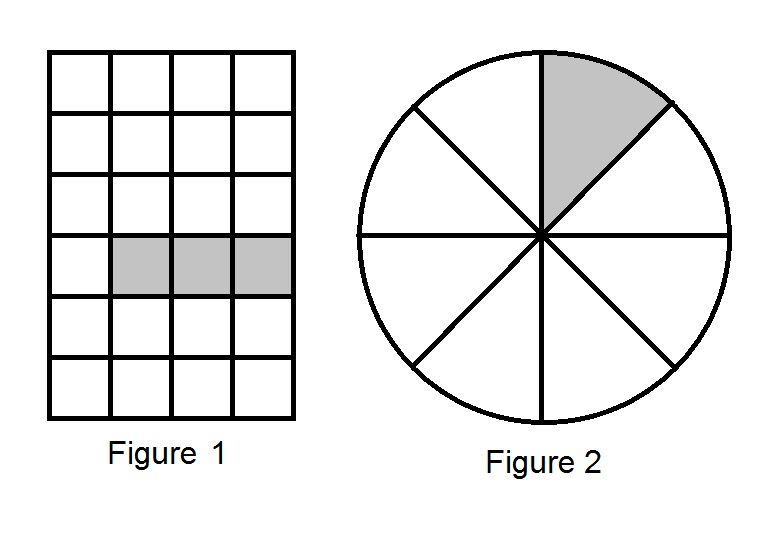Figure NOT drawn to scale.

Figure 1 and Figure 2 have the same area. The shaded portion of Figure 1 has area 64. What is the area of the shaded portion of Figure 2?Figure 1 is a rectangle divided into 24 squares of equal size; 3 of the squares are shaded, which means that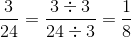of Figure 1 is shaded.
Figure 2 is a circle  divided into 8 sectors of equal size; 1 is shaded, which means thatof Figure 2 is shaded.